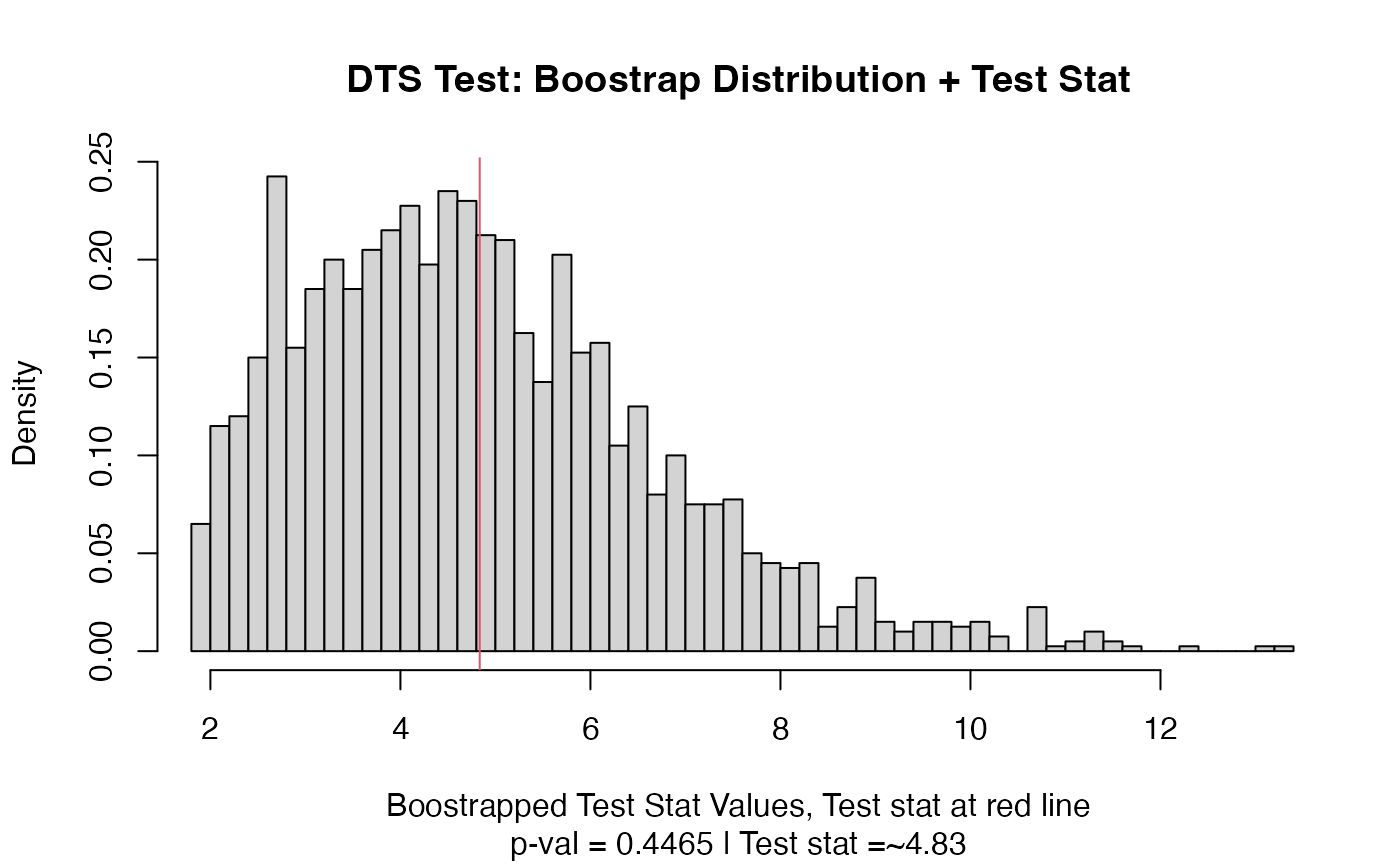Typically for now this will produce a histogram of the null distribution based on the bootstrapped values, with a vertical line marking the value of the test statistic.

## Usage

# S3 method for twosamples
plot(x, plot_type = c("boots_hist"), nbins = 50, ...)

## Arguments

x

an object produced by one of the twosamples *_test functions

plot_type

which plot to create? only current option is "boots_hist",

nbins

how many bins (or breaks) in the histogram

...

other parameters to be passed to plotting functions

## Value

Produces a plot

dts_test(), twosamples_class, combine.twosamples
out = dts_test(rnorm(10),rnorm(10,1))